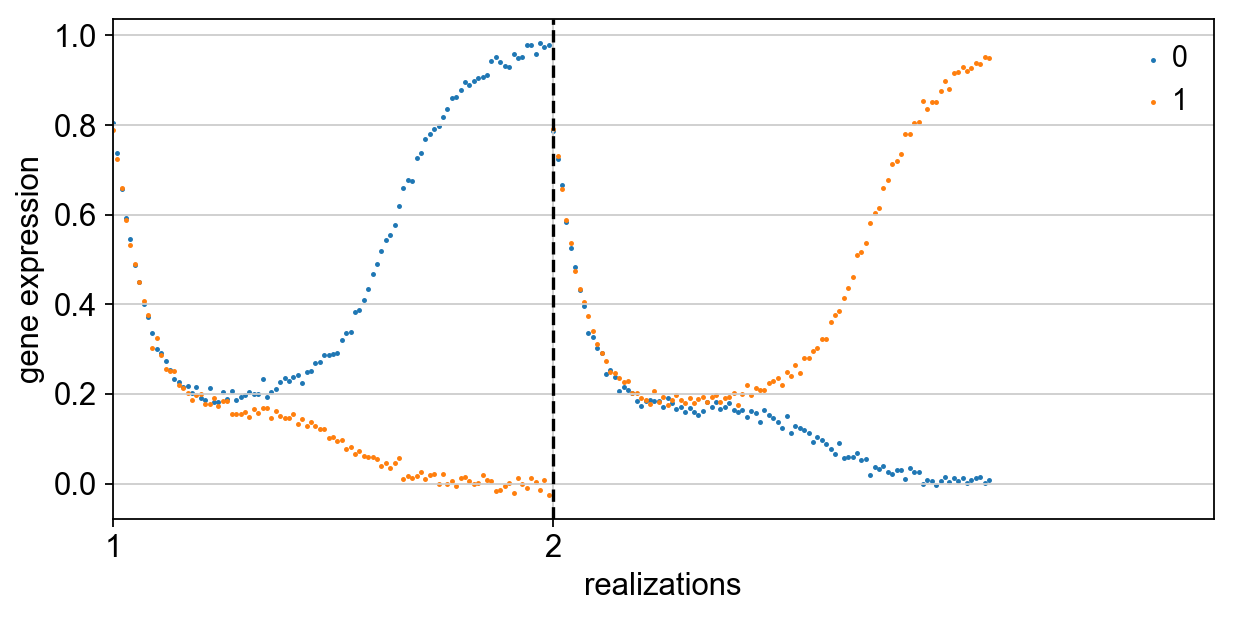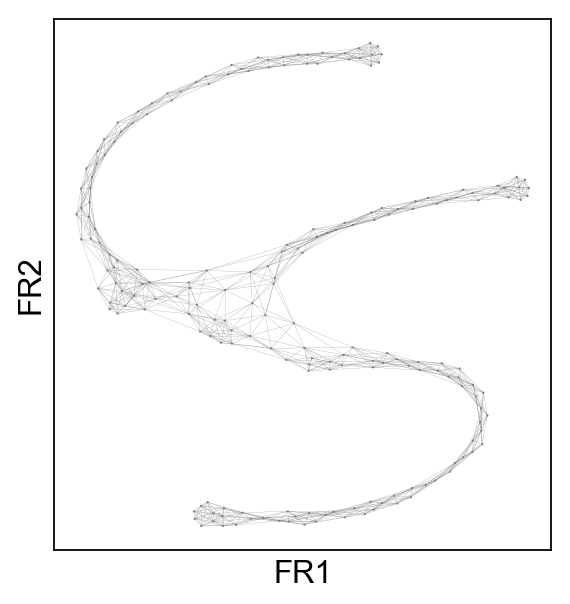First compiled on: November 25, 2017. Update March 28, 2017.

# Simulating a toggleswitch¶

In :
import scanpy.api as sc
sc.settings.verbosity = 3  # verbosity: errors (0), warnings (1), info (2), hints (3)
sc.settings.set_figure_params(dpi=80)  # low dpi (dots per inch) yields small inline figures
sc.logging.print_versions()

scanpy==1.0 anndata==0.5.8 numpy==1.14.1 scipy==1.0.0 pandas==0.22.0 scikit-learn==0.19.1 statsmodels==0.8.0 python-igraph==0.7.1 louvain==0.6.1


Simulating a simple toggleswitch (Gardner et al., Nature 2000).

In :
adata = sc.tl.sim('toggleswitch')

reading params file /Users/alexwolf/_hholtz/01_projects/1512_scanpy/scanpy/scanpy/sim_models/toggleswitch_params.txt
reading simulation results ./write/toggleswitch_sim/sim_000041.txt
Observation names are not unique. To make them unique, call .obs_names_make_unique.

In :
sc.pl.sim(adata)In :
sc.pp.neighbors(adata, n_neighbors=10)

computing neighbors
using data matrix X directly
finished (0:00:02.48) --> added to .uns['neighbors']
'distances', weighted adjacency matrix
'connectivities', weighted adjacency matrix

In :
sc.tl.draw_graph(adata)

drawing single-cell graph using layout "fr"
finished (0:00:00.34) --> added
'X_draw_graph_fr', graph_drawing coordinates (adata.obs)

In :
sc.pl.draw_graph(adata, edges=True, size=4)